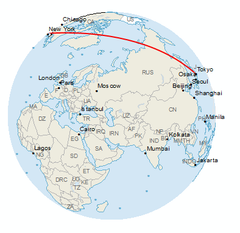# Trivia

What are Latitude and Longitude?

Latitude and longitude are part of the geographic coordinate system and they help us to map every point on the Globe. Latitude represents the coordinates going horizontally around the Globe whereas longitude represents the coordinates going vertically.

• Latitude is an invisible horizontal line that runs around the Earth from East to West. It begins at the Equator where its coordinates are 0°.
• The Equator divides the Earth into the Northern and Southern Hemispheres.
• Countries with a latitude of 0° include the Republic of the Congo, Uganda, Kenya, Indonesia and Brazil.
• The lines of latitude run parallel to the Equator in both the Northern and Southern Hemispheres, starting at 0° and counting to 90° North and 90° South.
• The North Pole has the latitude coordinate of 90°N (North) and the South Pole has the latitude coordinate of 90°S (South).
• Longitude is the invisible vertical line that runs around the Earth from North to South. The Prime Meridian is where its coordinates are 0°.
• Cities with a longitude of 0° include Greenwich and Cambridge (UK), Lleida (Spain) and Le Havre (France).
• Longitude divides the globe into the Eastern and Western Hemispheres along the Prime Meridian.
• The Prime Meridian originated in Greenwich, London which is why the longitude of 0° is set there. It runs through the UK, France, Africa and several seas.
• New York has a longitude coordinate of 74°W (West) and Cairo has a longitude coordinate of 31°E (East).
• Latitude and longitude coordinates are written as decimals with up to 4 decimal places to make sure they are accurate. For example, New York is 74.0059°W.
• If you combine a latitude coordinate with a longitude coordinate you can plot any point on the Earth. For example, 64.1265° N, 21.8174° W takes you to Reykjavik, the capital city of Iceland.

What is a Coordinate Reference System?

A reference ellipsoid is the mathematical model of the shape of the Earth with the major axis along the equatorial radius. A geographic coordinate system uses longitude and latitude expressed in decimal degrees.Cartographers write spherical coordinates (latitudes and longitudes) in two formats which are degrees-minutes-seconds (DMS) and decimal degrees. For degrees-minutes-seconds, minutes range from 0 to 60. For example, the geographic coordinate expressed in degrees-minutes-seconds for Tokyo, Japan is:

• Latitude: 35 degrees, 40 minutes, 48 seconds N or 35°40’48.00” N
• Longitude: 139 degrees, 46 minutes, 12 seconds E or 139°46’12.00’ E

You can also express geographic coordinates in decimal degrees. It’s just another way to represent that same location in a different format. For example, here is Tokyo, Japan in decimal degrees:

• Latitude: 35.6800° N
• Longitude: 139.7700° E